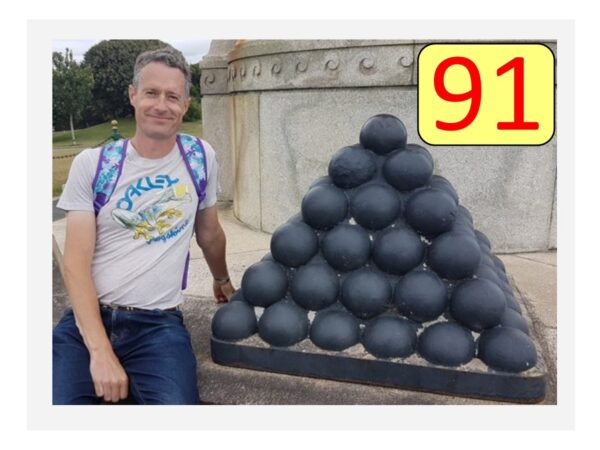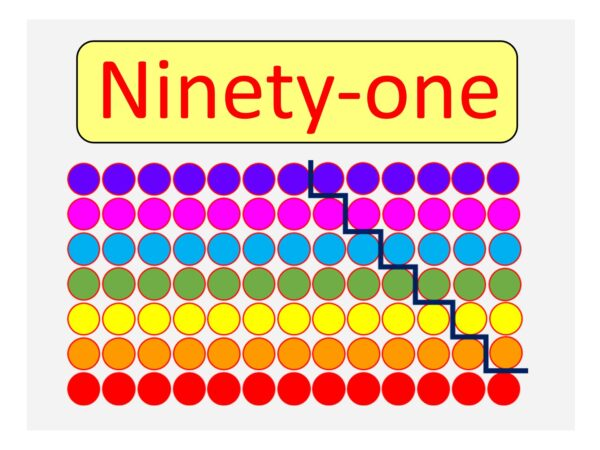07899 756585

# House of Maths School Workshops Primary & Secondary in Dorset & South - WHAT’S SPECIAL ABOUT THE NUMBER NINETY-ONE?

A WARM-UP: Quickly: is 91 a prime number or not?

Don’t think too hard, just your gut feeling: yes or no? I’ll return to this later, but first:

NINETY-ONE IS A TRIANGULAR NUMBER:
The Triangular numbers 1, 3, 6, 10, 15, 21, 28, 36, 45, 55, 66, 78, 91, … come up in lots of places, but at their simplest they are the number of snooker balls you would need for a triangle with, in this case 13 rows. Ninety-one is triangular because 91=1+2+3+4+5+6+7+8+9+10+11+12+13. So we could use the notation $91=T_{13}$ because it’s the thirteenth Triangular number.NINETY-ONE IS A SQUARE-BASED-PYRAMID NUMBER:
The SQUARE PYRAMIDAL NUMBERS 1, 5, 14, 30, 55, 91, … are the number of cannonballs you would need in a stack of nested squares with, in this case 6 rows. Ninety-one is Square-Based-Pyramidal because $91=1+4+9+16+25+36=1^2+2^2+3^2+4^2+5^2+6^2$.
So we could use the notation $91=P^4_6$ because it’s the 6th square-based pyramid number. The 4 after and slightly above the P says that it’s a 6th square-based pyramid number; the 6th triangular-based-pyramid number (yes that’s a thing too!) would be written $P^3_{6}$Interesting, don’t you think, that 91 is both triangular and pyramidal number? Amazingly it has been proven that the only other numbers with this property are 1, 55, and… wait for it … 208335. I find that fact AMAAAAZING!

BUT IS IT PRIME?
Well, 2 doesn’t go into it, neither does 3 or 5. It doesn’t appear in any of the times tables (up to 12×12), so it certainly looks prime at first glance. However, it is NOT prime, because 91=7×13. If you compare the rectangular image below with the triangular one above, you can see how to rearrange the 91 snooker balls into a 7×13 rectangle.This trick of rearranging the snooker balls in a big triangle to make a rectangle works for all the triangular numbers, so none of the triangular numbers (except for 3) are prime – they are all composite (meaning “not prime”).

ONE LAST TRICK:
91 also features in the following puzzle: what number comes next in this sequence? Can you see the answer? It’s very silly: if you can’t see it then try viewing the image upside down!